It’s December…it’s that time of year again…!
A collection of Mathematical Advent Calendars.

The Core Maths resources, now available on STEM Learning include many excellent Statistics resources. The resources are designed to enable students to use and apply statistics in unfamiliar contexts. There are several resources with large data sets available, very useful for students to get used to working with such data sets

Many of these resources could also be adapted for younger students, with my Year 10 students (UK age 14-15) I will use the data and parts of the questions from Averages and Spread: two which explores how to calculate and interpret different measures of location and dispersion.

If we look at the first question, for example, we have the data shown here. Students are required to calculate the mean, median, mode, interquartile range, range, variance and standard deviation (these last two we can save until Year 12).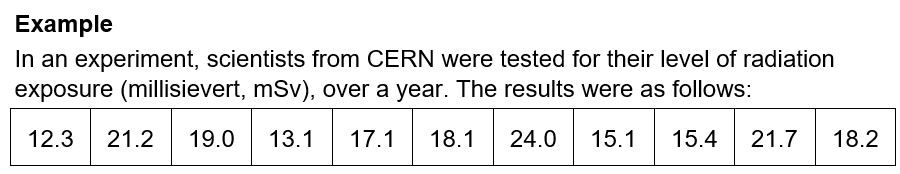I particularly like this interpretation question which we can use to give some context to the use of the upper quartile as here we have the upper quartile of 21.2 above the safe limit, suggesting that a quarter of scientists exceeded the safe limit.

The safe level for one year’s exposure is 20.0 mSv. Explain if the following statement is correct, using the data you have just calculated.

‘The scientists at CERN are working within the safe levels of radioactive exposure.’

Full solutions are given in the Teachers’ notes. The activity also includes questions on frequency distributions, one with grouped data.

We will also be able to review some charts and diagrams using these examples, drawing a box plot for the first example and a cumulative frequency chart for the grouped data example.

For some further useful resources, on TES we have a simple but effective exercise for students to practise drawing box plots byjhofmannmaths, a good example of a time-saving resource for teachers with ready drawn grids for students.

Looking at other resources by jhofmannmaths, I see many well-received resources; I like his simple but useful Expanding simple brackets mistakes which could be a useful Starter / Plenary (or anytime!).  Silly Suzie has made some errors! I appreciate resources like this with several copies to a page – very useful if you want to get a lesson started very promptly and have just changed room – it can be that the paper beats the technology!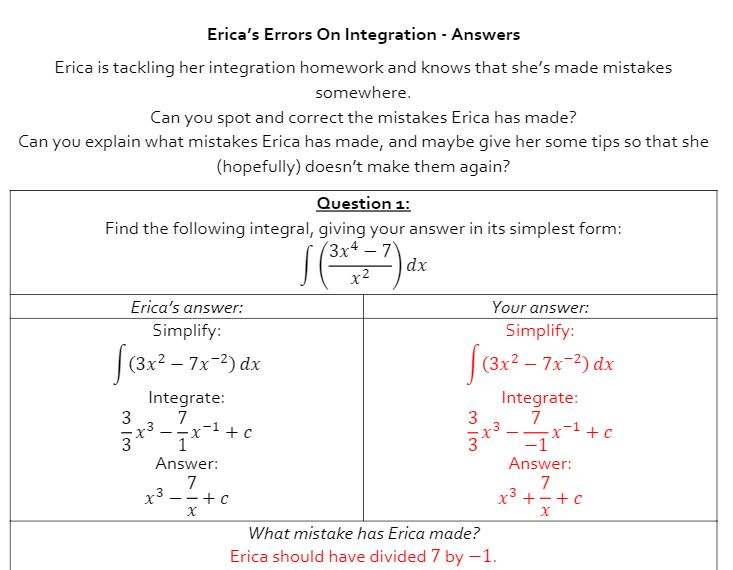Talking of silly Suzie reminds me of Erica and her errors by Andy Lutwyche. I used his  Erica’s Errors on Integration very successfully with Year 12 this week, many of whom made the same mistakes as Erica. We had some great discussions on how easy it is to make such errors and they seem to believe me more than ever that having a look at the graph is a really good idea!

# Integration – Desmos & WolframAlpha

With my year 12 students (UK age 16-17) we have been looking at definite Integration. Desmos and WolframAlpha are both excellent for checking work and by using the technology we have a very clear visual representation adding to our understanding.

One of the homework questions for my students involves finding the total shaded area bounded by f(x) = x4−3x3−4x2+12x, the x-axis, the line x=−1 and the line x=3.

We could use WolframAlpha for a quick check. I like the visual representation showing students clearly that they are dealing with areas above and below the x-axis.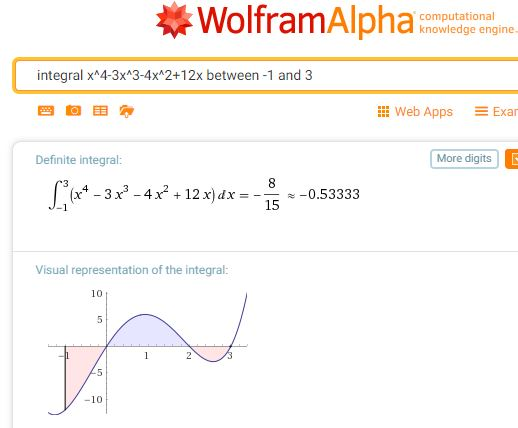Scrolling down the page we see that this query also returns the indefinite integral.For the total shaded area, students could change the limits of the query to evaluate each section.

Or we could turn to the excellent Desmos where we can very simply change the limits.

If you are unfamiliar with Integration with Desmos, turn to Learn Desmos: Integrals.
Note you can explore the graph shown in the video.

A version of this post for students which also includes links to some useful notes and examples can be found on Mathematics for Students.# Math is fun!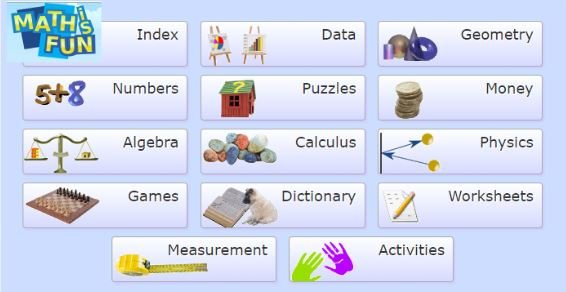A favourite site – Mathisfun! is a treasure trove of resources.
There are clear indices; the Index from the Home page takes you to the very useful Index by Year and Subject. Explanations and examples are very clear and each section has additional questions to try with solutions. Look for example at the Algebra Index and choose any topic to see the very clearly presented information and activities.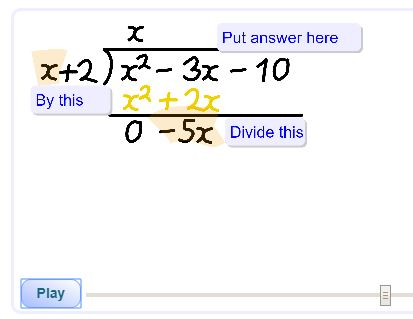Polynomial Long Division

You can also use the following pages to search :

I often refer to this site and know that students like the clear presentation. I have used everything from Balance when Adding and Subtracting with younger students to working out the inverse of a 3×3 Matrix with the Sixth Form and lots in between! The Dictionary too is very helpful.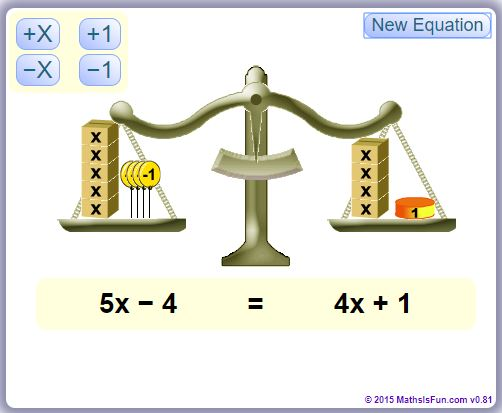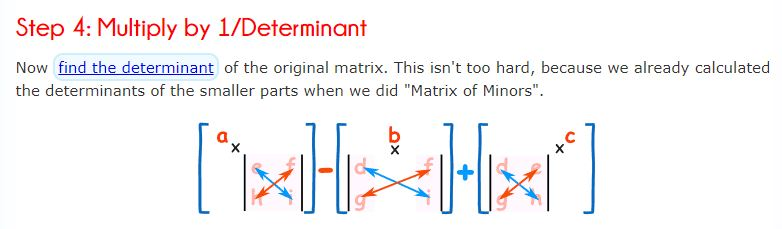Thinking about my current teaching, with Year 12 we are looking at the Binomial Expansion, we can see some clear illustrations here including a rather nice illustration for (a+b)3.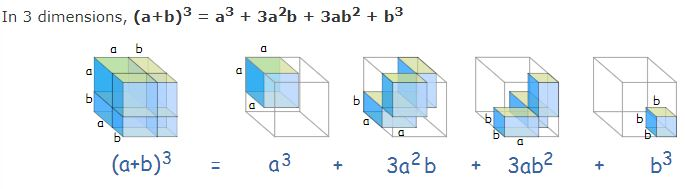With Year 10, having met Surds for the first time this year, some additional examples will be helpful; as mentioned above – each section has a quiz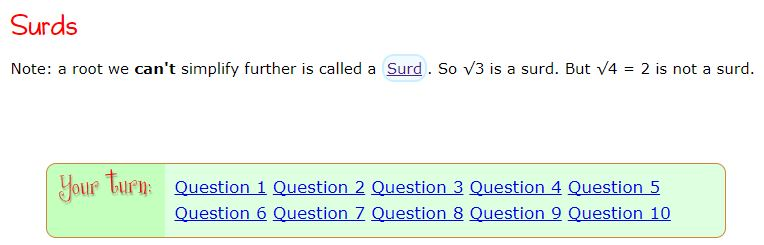My Further Mathematicians are studying Complex Numbers, some rather nice illustrations can be found here for them.